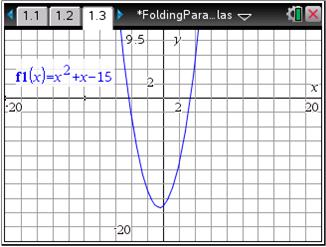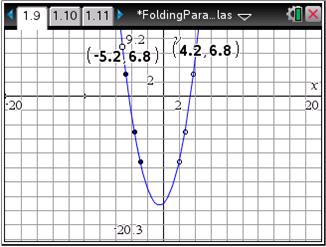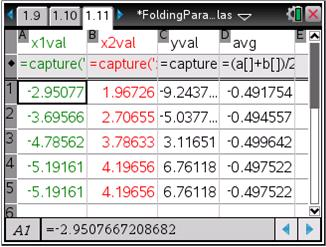# Activities

••• ##### Subject Area

• Math: Algebra I: Quadratic Functions

• ##### Author9-12

20 Minutes

• ##### Device
•TI-Nspire™ CX/CX II
•TI-Nspire™ CX CAS/CX II CAS
• TI-Nspire™
• TI-Nspire™ CAS
• ##### Software

TI-Nspire™
TI-Nspire™ CAS

3.2

## Folding Parabolas

#### Activity Overview

In this activity, students graph a quadratic function and investigate its symmetry by choosing pairs of points with the same y-value. They then calculate the average of the x-values of these points and discover that not only do all the points have the same x-value, but the average is equal to the x-value of the vertex.

#### Key Steps

•This activity enables students to investigate the axis of symmetry of a quadratic.

Students graph a quadratic equation and examine it.

•A page is set up for students to gather data from a graph of a quadratic function.

Students will use the manual data capture to record the x-coordinates with the same y-values.

•Students use a formula to calculate the average of two x-coordinates with the same y value.

After capturing the points and finding the averages of their x-values, students will use the data to investigate and form a hypothesis about the axis of symmetry and the mean of two x-values.

At the end of this activity, students will be able to use the axis of symmetry to find the coordinates of the vertex.